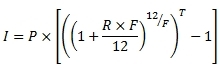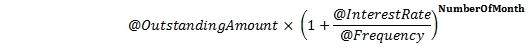# SQL Server amortization for bullet loans

Bullet

Updated: 18 September 2014

Use the table-valued function Bullet to return the cash flow schedule for a loan with a single payment of principal and interest at maturity. Only 2 rows are returned: one for the commencement of the loan and one for the maturity date of the loan.
The interest payment is calculated as:Where:

 I = InterestPayment P = @OutstandingAmount R = @InterestRate F = @Frequency T = Time in years

Syntax
SELECT * FROM [wct].[Bullet](
<@OutstandingAmount, float,>
,<@InterestBasis, nvarchar(4000),>
,<@InterestRate, float,>
,<@Frequency, int,>
,<@MaturityDate, datetime,>
,<@ReferenceDate, datetime,>)
Arguments
@OutstandingAmount
the principal amount of the loan. @OutstandingAmount is an expression of type float or of a type that can be implicitly converted to float.
@InterestBasis
the day count convention used to calculate the interest amount. @InterestBasis can be 30/360, Actual/360, Actual/365, or Actual/Actual. @InterestBasis is an expression of the character string data type category.
@InterestRate
the annual rate of interest for the loan. @InterestRate is an expression of type float or of a type that can be implicitly converted to float
@Frequency
the number of months in a regular interest payment. @Frequency is an expression of type int or of a type that can be implicitly converted to int.
@MaturityDate
the maturity date of the loan. @MaturityDate is an expression that returns a datetime or smalldatetime value, or a character string in date format.
@ReferenceDate
the start date of the loan. @ReferenceDate is an expression that returns a datetime or smalldatetime value, or a character string in date format.
Return Type
RETURNS TABLE (
[Period] [int] NULL,
[PrincipalPayment] [float] NULL,
[InterestPayment] [float] NULL,
[CashFlow] [float] NULL,
[OutstandingExposure] [float] NULL,
[CapitalAmountInDebt] [float] NULL,
[TotalExposure] [float] NULL,
[NumberOfMonth] [int] NULL,
[PaymentDate] [datetime] NULL,
[GraceInterest] [float] NULL,
[InterestRate] [float] NULL
)

 Column Description Period A reference number uniquely identifying a row in the resultant table. PrinicpalPayment The amount of the principal payment. InterestPayment The amount of the interest payment. CashFlow The amount of the cash flow. OutstandingExposure When Period = 0, @OutstandingAmount. When Period = 1, @OutstandingAmount + InterestPayment. CapitalAmountInDebt When Period = 0, @OutstandingAmount. When Period = 1, 0 TotalExposure See below. NumberOfMonth The number of months between the @ReferenceDate and the PaymentDate. PaymentDate The end-of-month date when the payment occurs. GraceInterest 0 InterestRate The interest rate from the @ReferenceDate to the @MaturityDate. See formula above.

Remarks
·         The PaymentDate for all rows is generated as the last day of the month.
·         For Period = 0, PrincipalPayment, InterestPayment, CashFlow, NumberOfMonth, GraceInterest, and InterestRate are set to 0.
·         The time value (see formula above) is calculated using the day-count convention specified by @InterestBasis:
o        For Actual/360 it is the number of days between the 2 PaymentDate values divided by 360 .
o        For Actual/365 it is the number of days between the 2 PaymentDate values divided by 365.
o        For Actual/Actual it is the number of days between the 2 PaymentDate values divided by the number of days in the year of the later PaymentDate.
o        For 30/360 is the number of months between the 2 PaymentDate values divided by 12.
·         If @InterestBasis is NULL then @InterestBasis = 30/360
·         If @InterestBasis is not 30/360, ACTUAL/360, ACTUAL/365, or ACTUAL/ACTUAL then an error message will be generated.
·         If @Frequency is NULL then @Frequency = 1
·         If @InterestRate is NULL then @InterestRate = 0
·         If @ReferenceDate is NULL then @ReferenceDate = GETDATE()
·         If @MaturityDate is NULL then @MaturityDate = GETDATE()
·         TotalExposure is calculated as:Examples
SELECT
*
FROM wct.Bullet(
6000000             --@OutstandingAmount
,'Actual/360'        --@InterestBasis
,.07                 --@InterestRate
,3                   --@Frequency
,'2015-07-05'        --@MaturityDate
,'2014-06-30'        --@ReferenceDate
)
This produces the following result (which has been reformatted for ease of viewing).

 Period Principal Payment Interest Payment Cash Flow Outstanding Exposure Capital Amount In Debt Total Exposure Number Of Month Payment Date Grace Interest Interest Rate 0 0.00 0.00 0.00 6000000.00 6000000.00 6000000.00 0 2014-06-30 0.00 0.000000 1 6000000.00 475938.10 6475938.10 6475938.10 0.00 6471270.64 13 2015-07-31 0.00 0.079323

### SupportCopyright 2008-2023 Westclintech LLC         Privacy Policy        Terms of Service